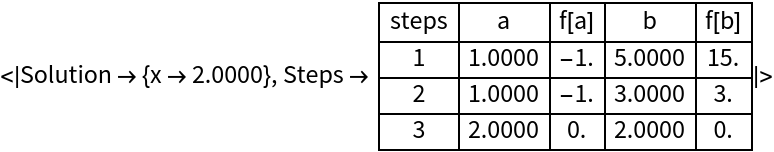#Function Repository Resource:

# BisectionMethodFindRoot

Determine the root of an equation using the bisection method

Contributed by: Jason Martinez
 ResourceFunction["BisectionMethodFindRoot"][f,{x,xa,xb},tol,n] searches for a numerical root of f between the points xa and xb using tol digits and up to n steps. ResourceFunction["BisectionMethodFindRoot"][lhs⩵rhs,{x,xa,xb},tol,n] searches for a numerical solution to the equation lhs==rhs. ResourceFunction["BisectionMethodFindRoot"][f,{x,xa,xb},tol,n,property] returns a property of the search for the root of f.

## Details and Options

BisectionMethodFindRoot supports two options for property:
 "Solution" return the root of f "Steps" return a table of steps taken to reach the root
"PropertyAssociation" can be used to return an Association of the properties.
BisectionMethodFindRoot terminates when the result is correct to the requested tolerance or the maximum number of steps has been taken, whichever comes first.

## Examples

### Basic Examples

Find the root of an expression using the bisection method:

 In:=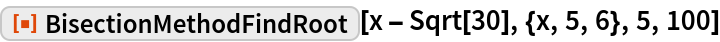Out=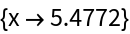Determine the steps to find the root of an equation:

 In:=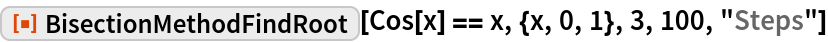Out=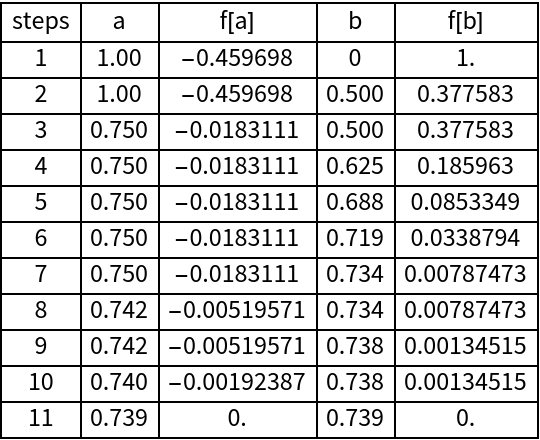### Scope

Get the property association of a bisection search:

 In:=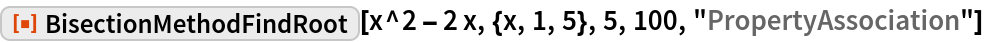Out=